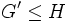# Abelian-quotient subgroup

This article defines a subgroup property: a property that can be evaluated to true/false given a group and a subgroup thereof, invariant under subgroup equivalence. View a complete list of subgroup properties[SHOW MORE]

## Definition

### Symbol-free definition

A subgroup of a group is termed an Abelian-quotient subgroup if it satisfies the following equivalent conditions:

### Definition with symbols

A subgroup$H$ of a group [itex]G[/itex] is termed an Abelian-quotient subgroup if it satisfies the following equivalent conditions:

•$G' \le H$ where [/itex]G' = [G,G][/itex] is the commutator subgroup of$G$
•$H$ is normal in$G$ and$G/H$ is an Abelian group